# K-D树结构原理与实例详解，可用于机器学习k邻近算法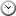2020-02-21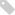数据结构, AI机器学习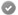浏览次数：1889

KD树是二叉树数据结构，同时也运用了排序二叉树的思想，主要用于范围式的查找，KD树最擅长的是能够对多维属性进行查找，此数据结构与K邻近分类算法搭配使用，能很好的实现对未知事物的分类工作，这里有些知识不再重复，例如：在多维空间里，如何计算连个点之间的距离？什么是K邻近分类算法？如果这些都没懂的话，希望先看另一篇文章，“K邻近分类算法详解”。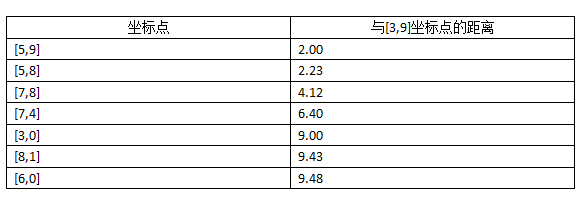1 首先选定某个轴向作为初始划分轴，在这个轴向上对数据进行排序，取中间位置的数据作为树节点，在这轴向上，小于节点轴向值的一律放左边，大于或等于的一律放右边（其实是将数据对半划分）。
2 然后选下一个轴向，继续对剩余的数据进行划分，根据步骤1的规则，将数据对半划分。
3 反复循环1和2步骤，直到没有数据为止。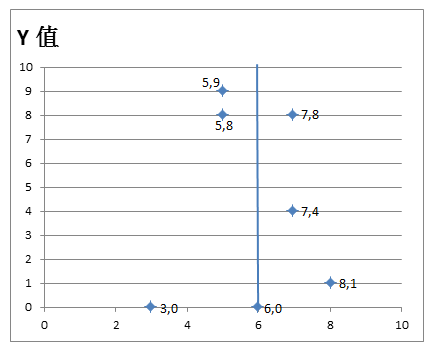-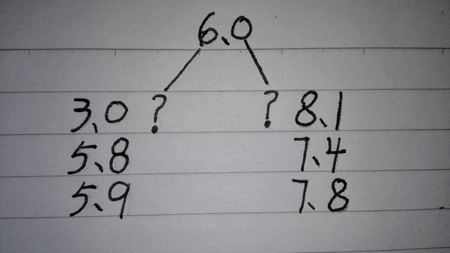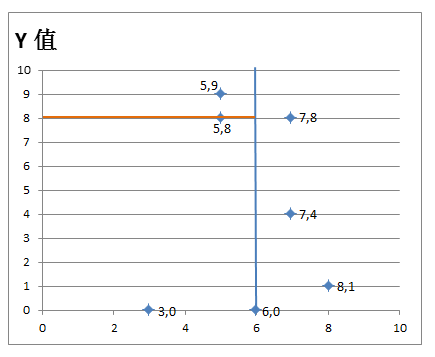-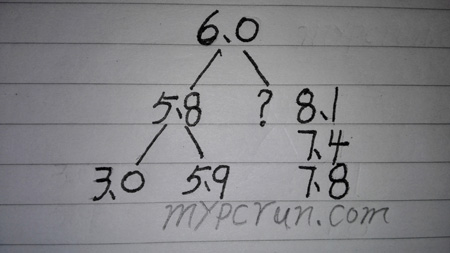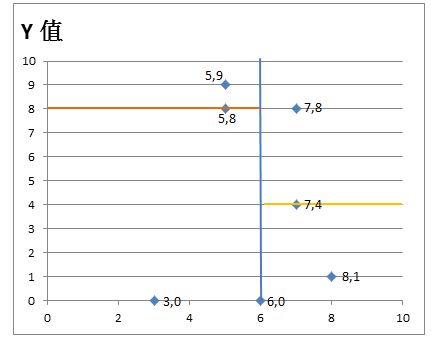-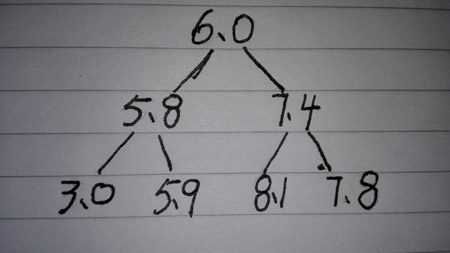1 每到一个节点，都计算跟查询点的距离，如果距离比当前最近距离还要小，就将此值设置为当前最近距离

2 在当前节点的划分轴上，计算当前节点与查询点在此轴上的值的差，然后将差变成绝对值，这个值我将其称为轴距差，然后根据以下三种情况分别处理：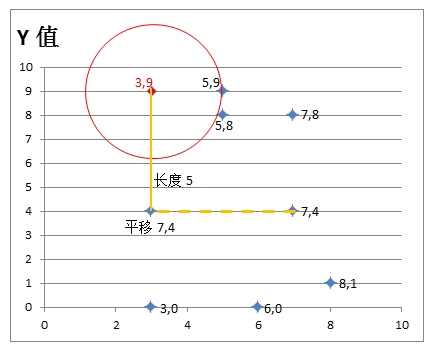5=3（查询点的X轴值）+ 2（与查询点的距离）
1=3（查询点的X轴值）- 2（与查询点的距离）

11=9（查询点的Y轴值）+ 2（与查询点的距离）
7=9（查询点的Y轴值）- 2（与查询点的距离）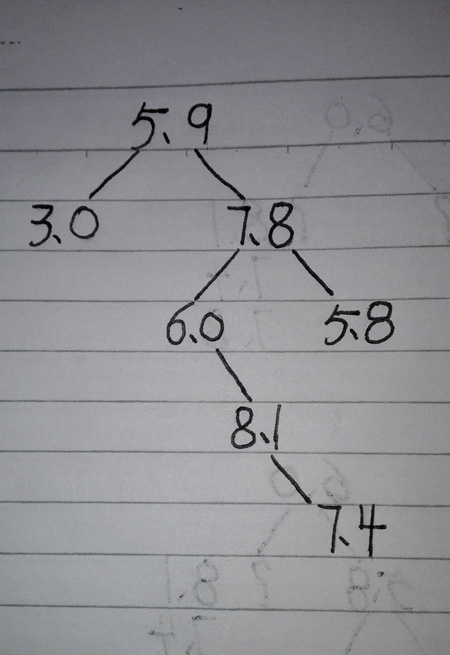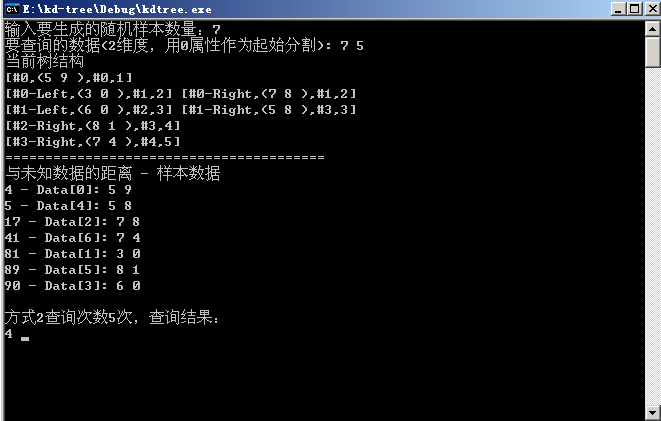#include <stdlib.h>
#include <memory.h>
#include <stdio.h>
#include <iostream.h>
#include <time.h>
#include <math.h>

// 三维数组，可改成多维数组 aryValue,aryValue等等
typedef int aryValue;

// 树节点结构
typedef struct _TreeNode{
aryValue NodeKey; // 节点值
int Depth; // 子树的最大深度值
_TreeNode* left; // 左子节点
_TreeNode* right; // 右子节点
}TTreeNode, *PTreeNode;

// 对数组里的某个属性计算方差
double GetTheVariance(aryValue* lparyValue, int aryValueCount, int x)
{
double floatResult=0;

if (!lparyValue) return floatResult;

// 先获取总值
unsigned int D=0;
int N=0;
while (N < aryValueCount){
D=D+lparyValue[N][x];
N++;
}

// 再取平均值
int P=D/aryValueCount;

// 累计每一个数跟平均值的差的平方
unsigned int T=0;
unsigned int H=0;
N=0;
while (N < aryValueCount){
int K=lparyValue[N][x] - P;
T=T+K*K;
N++;
// 为防止溢出，累计大于30亿的数值，将提前计算一次，但会带来微小的误差
if (T > 3000000000){
H=H+T/aryValueCount;
T=0;
}
}

if (T > 0) H=H+T/aryValueCount;

floatResult=sqrt(H);

return floatResult;
}

// 计算两个点的距离的平方
int TowPointDistance(aryValue p1,aryValue p2)
{
int intResult=0;

int S=sizeof(aryValue) / sizeof(int);

int N=0;
while (N < S){
int A=p1[N] - p2[N];
intResult=intResult+A*A;
N++;
}

// 注释掉这句话，是因为开方计算耗时，实际上，程序主要是利用距离的长度做对比，没必要进行开方
// intResult=sqrt(intResult);

return intResult;
}

// 将数组按照某个属性进行排序
void SortAryData(aryValue* aryData, int aryDataCount, int x)
{
int L=sizeof(aryValue);

int N=aryDataCount;
while (N > 0){
int M=0;
while (M < N){
int T=aryData[M][x];
int K=M+1;
if (K < N){
if (T > aryData[K][x]){
aryValue S;
memcpy(&S,aryData[M],L);
memcpy(aryData[M],aryData[K],L);
memcpy(aryData[K],&S,L);
}
}
M++;
}
N--;
}
}

/*

*/
typedef struct _ListData{
int D; // 距离
int indx; // 原始数据所在数值的原位置
void* pdata; // 指向数据的指针
_ListData* next; // 指向下一个指针
}TListData, *PListData;

void getNearNode_2(aryValue* lparyValue,int lparyValueCount,aryValue unKnowData, int K)
{
printf("与未知数据的距离 - 样本数据\r\n");

PListData FirstListData=0;

int S=sizeof(aryValue) / sizeof(int);
int N=0;
while (N < lparyValueCount){
// 两点之间的距离
int D=TowPointDistance(unKnowData,lparyValue[N]);

PListData lpListData=new TListData;
lpListData->D=D;
lpListData->indx=N;
lpListData->pdata=lparyValue[N];
lpListData->next=0;

if (FirstListData){
PListData P=FirstListData;
PListData P2=0;
while (P){
if (lpListData->D <= P->D){
lpListData->next=P;
if (P2){
P2->next=lpListData;
}
else{
FirstListData=lpListData;
}
break;
}
P2=P;
P=P->next;
}
}
else{
FirstListData=lpListData;
}

N++;
}

N=0;
PListData P=FirstListData;
while (P){
N++;
// printf("%lf - ",sqrt(P->D));
printf("%d - ",P->D);
aryValue* pData=(aryValue*)P->pdata;
printf("Data[%d]:",P->indx);
int T=0;
while (T < S) printf(" %d",(*pData)[T++]);
printf("\r\n");
P=P->next;
if (N > 30){
printf("只输出前30位距离最近的数据\r\n");
break;
}
}

}

/*

lpTreeRootNode: 根节点
Depth: 节点所在层
lparyValue: 存储数据样本的缓冲区
lparyValueCount: 数据样本数量
Dimension: 维度
*/
void KDTreeCreate(PTreeNode* lpTreeRootNode,int Depth,aryValue* lparyValue,int lparyValueCount,int Dimension)
{
Depth++;

// 将集合排序
if (lparyValueCount > 1) SortAryData(lparyValue,lparyValueCount,Dimension);

// 计算中间位置值
int Median=lparyValueCount / 2;

// 但是还不行，因为某维度的数值可能存在多个相同的数值
int C=lparyValue[Median][Dimension];
int N=Median;
while (N > 0){
N--;
if (lparyValue[N][Dimension] >= C){
Median--;
}
else{
break;
}
}

// 得到的中间值成为根节点
PTreeNode lpTreeNode=new TTreeNode;
lpTreeNode->left=0;
lpTreeNode->right=0;
lpTreeNode->Depth=Depth;
memcpy(&lpTreeNode->NodeKey,lparyValue[Median],sizeof(aryValue));
*lpTreeRootNode=lpTreeNode;

// 从下一维度数据开始划分
Dimension++;
if (Dimension >= sizeof(aryValue) / sizeof(int)) Dimension=0;

// 从此数组的中间位置，分成两组

// 小于中间值的成为左边一组
int LeftAryValueCount=Median;
if (LeftAryValueCount > 0){
aryValue* LeftAryValue=lparyValue;
KDTreeCreate(&lpTreeNode->left,Depth,LeftAryValue,LeftAryValueCount,Dimension);
}

// 大于中间值的成为右边一组
int RightAryValueCount=lparyValueCount-Median-1;
if (RightAryValueCount > 0){
aryValue* RightAryValue=lparyValue+Median+1;
KDTreeCreate(&lpTreeNode->right,Depth,RightAryValue,RightAryValueCount,Dimension);
}
}

// 将最近距离值保存进查找结果数组
void AddNearDistance(PListData* ListData,int Distance,PTreeNode lpTreeRootNode,int K)
{
// 找出存储结果数组中的最大值的下标
int ListDataCount=0;
int MaxDistance=0;
int MaxIndx=0;
PListData lpListData=*ListData;
while (lpListData){
if (lpListData->D > MaxDistance){
MaxDistance=lpListData->D;
MaxIndx=ListDataCount;
}
lpListData=lpListData->next;
ListDataCount++;
}

// 数组最多只存储一定量的数据
if (ListDataCount < K){
PListData NewListData=new TListData;
NewListData->D=Distance;
NewListData->indx=0;
NewListData->pdata=lpTreeRootNode;
NewListData->next=*ListData;
*ListData=NewListData;
}
else{
// 大于 K 个，看当前距离值是否小于数组里的最大值，如果大于，替换
if (Distance < MaxDistance){
int T=0;
PListData lpListData=*ListData;
while (lpListData){
if (T==MaxIndx){
lpListData->D=Distance;
lpListData->pdata=lpTreeRootNode;
break;
}
lpListData=lpListData->next;
T++;
}
}
}

}

/*

lpTreeRootNode: 树根节点
SearchData: 要查询的数据
Dimension: 查询维度
MinD: 当前最近距离

// 下面的参数在实际项目中可有可无，自己设计更好的数据保存方法
ListData: 用于保存最靠近查询点的几个节点
Se: 用于统计递归执行次数
K: 保存多少个最近的距离
*/
int KDTreeSearchNode(PTreeNode lpTreeRootNode,aryValue SearchData,int Dimension,PListData* ListData,int* MinD,int *Se,unsigned int K)
{
int intResult=0;

if (!lpTreeRootNode || !SearchData) return intResult;

// 累计执行次数
*Se=*Se+1;

// 下一个节点所代表的属性划分点
int L=sizeof(aryValue) / sizeof(int);
int NextDimension=Dimension+1;
if (NextDimension >= L) NextDimension=0;

// 当前节点的属性值
int NodeDimensionValue=lpTreeRootNode->NodeKey[Dimension];
// 查询点的属性值
int SearchDimensionValue=SearchData[Dimension];

// 计算当前节点与查询点的距离
int Distance=TowPointDistance(lpTreeRootNode->NodeKey,SearchData);

// 将当前点的距离放入数组里

// 如果当前节点距离小于等于最短距离，那么将当前点设置为最近点，MinD 值为负数表示无限大
if ((*MinD < 0) || (Distance <= *MinD)) *MinD=Distance;

// 当前节点对应的维度，与查询点对应的维度的距离
int D=SearchDimensionValue - NodeDimensionValue;
D=D*D;

// 查看左右子节点是否有在范围内
if (D <= *MinD){
if (lpTreeRootNode->left) KDTreeSearchNode(lpTreeRootNode->left,SearchData,NextDimension,ListData,MinD,Se,K);
if (lpTreeRootNode->right) KDTreeSearchNode(lpTreeRootNode->right,SearchData,NextDimension,ListData,MinD,Se,K);
}
else{
if ((NodeDimensionValue < SearchDimensionValue) && lpTreeRootNode->right)
KDTreeSearchNode(lpTreeRootNode->right,SearchData,NextDimension,ListData,MinD,Se,K);

if ((NodeDimensionValue > SearchDimensionValue) && lpTreeRootNode->left)
KDTreeSearchNode(lpTreeRootNode->left,SearchData,NextDimension,ListData,MinD,Se,K);
}

return intResult;
}

/*

*/

{
if (!*RootNode){
PTreeNode lpTreeNode=new TTreeNode;
lpTreeNode->left=0;
lpTreeNode->right=0;
lpTreeNode->Depth=1;
memcpy(&lpTreeNode->NodeKey,newData,sizeof(aryValue));
*RootNode=lpTreeNode;
return;
}

// 下一个节点所代表的属性划分点
int L=sizeof(aryValue) / sizeof(int);
int NextDimension=Dimension+1;
if (NextDimension >= L) NextDimension=0;

PTreeNode lpTreeRootNode=*RootNode;

// 当前节点的属性值
int NodeDimensionValue=lpTreeRootNode->NodeKey[Dimension];
// 查询点的属性值
int newDimensionValue=newData[Dimension];

if (newDimensionValue < NodeDimensionValue){
// 新数据的属性值小于当前节点的属性值，进入左子树
if (lpTreeRootNode->left){
}
else{
}
}
else{
// 新数据的属性值大于或等于当前节点的属性值，进入右子树
if (lpTreeRootNode->right){
}
else{
}
}

PTreeNode lpTreeNode=new TTreeNode;
lpTreeNode->left=0;
lpTreeNode->right=0;
lpTreeNode->Depth=lpTreeRootNode->Depth + 1;
memcpy(&lpTreeNode->NodeKey,newData,sizeof(aryValue));
}
}

// 将整颗树的数据显示出来
int FValue; // 父节点的编号
int Depth; // 层次
int location; // 位置，1 左边；2 右边
_TreeNode* TreeNode; // 指向一个树的节点

void PrintfTree(PTreeNode RootNode)
{
// 采用广度优先遍历

if (!RootNode) return;

printf("当前树结构\r\n");

int NodeCount=0;

int N=0;
printf("\r\n");
}

char strLocation={0};

int T=0;
while (T < (sizeof(aryValue) / sizeof(int))) printf("%d ",lpNodeLink->TreeNode->NodeKey[T++]);

// 将树节点的左右子节点加入队列后面

}

}

}
else{
}

break;
}
}
}

NodeCount++;
}

/*

*/
}

printf("\r\n========================================\r\n");
}

int main()
{
// 随机生成若干个多维数据，作为样本
printf("输入要生成的随机样本数量：");
int lparyValueCount=0;
cin>>lparyValueCount;
if (lparyValueCount <= 0) return 0;

aryValue* lparyValue=new aryValue[lparyValueCount];

int MaxNumber=9000;
srand(time(0));
int L=sizeof(aryValue) / sizeof(int);
int N=0;
while (N < lparyValueCount){
int T=0;
while (T < L) lparyValue[N][T++]=rand()%MaxNumber;
N++;
}

// 对样本数据，计算每个维度的方差，最终选择方差最大的维度，作为切分点
N=0;
double MinF=0;
int SelectN=0;
while (N < L){
double F=GetTheVariance(lparyValue,lparyValueCount,N);
if (F > MinF){
SelectN=N;
MinF=F;
}
N++;
}

// 再随机生成一个未知数据
printf("要查询的数据(%d维度，用%d属性作为起始分割):",L,SelectN);
aryValue unKnowData={0};
N=0;
while (N < L){
unKnowData[N]=rand()%MaxNumber;
printf(" %d",unKnowData[N]);
N++;
}
printf("\r\n");

// 构造KD树
PTreeNode TreeRootNode=0;
KDTreeCreate(&TreeRootNode,0,lparyValue,lparyValueCount,SelectN);

// 往KD树添加数据
// aryValue newData={1,2,3};

// 显示树结构
// PrintfTree(TreeRootNode);

// 采用最原始的方法，逐个计算对比，得出的结果按距离从小到大进行排序
getNearNode_2(lparyValue,lparyValueCount,unKnowData,10);

// 查询KD树
PListData ListData=0;
int MinD=-1;
int Se=0;
KDTreeSearchNode(TreeRootNode,unKnowData,SelectN,&ListData,&MinD,&Se,5);

// 打印查询结果
printf("\r\n方式2查询次数%d次，查询结果：\r\n",Se);
while (ListData){
printf("%d ",ListData->D);
ListData=ListData->next;
}

delete[] lparyValue;

int inputvalue=0;
cin>>inputvalue;

return 0;
}

Good good study, day day up!

Email

2023 mypcrun.com